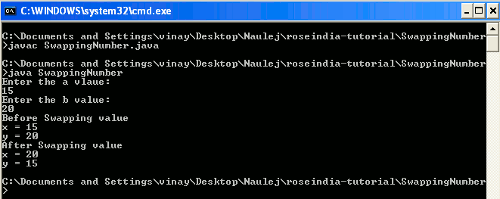Tutorials

# Swapping of two numbers in java

In this example we are going to describe swapping of two numbers in java without using the third number in java. We have used input.nextInt() method of Scanner class for getting the integer type values from the command prompt.

In this example we are going to describe swapping of two numbers in java without using the third number in java. We have used input.nextInt() method of Scanner class for getting the integer type values from the command prompt.

## Swapping of two numbers in java

In this example we are going to describe swapping of two numbers in java  without using the third number in java. We have used input.nextInt() method of Scanner class for getting the integer type values from the command prompt. The swapping of two numbers is based on simple arithmetic operation that has been described below in simple steps. Same logic has been applied in the programming as well.

Following is the method how to number are swapped:-

First we take two variable "a" and "b".

initially a = 15 and b= 20.

Step 1     a= a+b given a=15+20
a=35

Step 2    b= a-b given b= 35-20
b=15

Step 3    a = a-b given a= 35-15
a =20

Finally   a=20 and b=15

Example

```package SwappingNumber;
import java.util.Scanner;

public class SwappingNumber {
public static void main(String args[])
{
int a, b, num;
System.out.println("Enter the a vlaue:");
Scanner in = new Scanner(System.in);
a = in.nextInt();
System.out.println("Enter the b value:");
b = in.nextInt();

System.out.println("Before Swapping value\nx = "+a+"\ny = "+b);

num = a;
a = b;
b = num;

System.out.println("After Swapping value\nx = "+a+"\ny = "+b);
}
}```

Output